WEBINAR
Magnetic Levitation for Energy Harvesting
Thursday, June 22, 2023
Time
SESSION 1
SESSION 2
CEST (GMT +2)
03:00 PM
08:00 PM
EDT (GMT -4)
09:00 AM
02:00 PM
HOME / Applications / 3-phase transformer

# 3-phase transformer

Used Tools:A transformer (Figure 1) is an electrical device that transfers electrical energy between two or more circuits through electromagnetic induction. Electromagnetic induction produces an electromotive force within a conductor which is exposed to time varying magnetic fields. Transformers are used to increase or decrease the alternating voltages in electric power applications.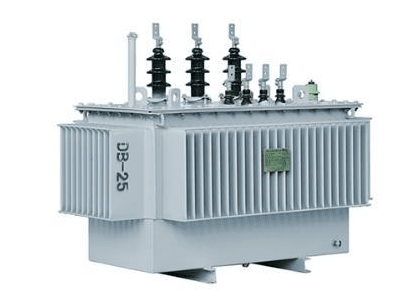Figure 1 - Power Transformer

Different types of losses are caused in a real operating transformer
Core losses, collectively called magnetizing current losses, consist of :

- Hysteresis losses due to nonlinear application of the voltage applied in the transformer core, and
- Eddy current losses due to joule heating in the core that are proportional to the square of the transformer's applied voltage.

The windings in a real transformer have finite non-zero resistances and inductances associated with:
- Joule losses due to resistance in the primary and secondary windings
- Leakage flux that escapes from the core and passes through one winding only resulting in primary and secondary reactive impedance.

By Faraday's Law of induction transformer EMFs vary according to the derivative of flux with respect to time.  The ideal transformer's core behaves linearly with time for any non-zero frequency. Flux in a real transformer's core behaves non-linearly in relation to magnetization current as the instantaneous flux increases beyond a finite linear range resulting in magnetic saturation associated with increasingly large magnetizing current, which eventually leads to transformer overheating.

Using EMS to simulate a transform's 3D model can help industrials  to reduce these  losses and thus increase the transformer’s efficiency and lifetime.

## Description

The example presented here is a three-phase transformer (Figure 3). The primary coils for each phase are characterized by (300 turns, 1 A/turn, 0 degrees), (300 turns, 1 A/turn, 120 degrees), and (300 turns, 1 A/turn, 240 degrees), respectively. The secondary windings are short-circuited. The windings are made of copper, and the core is composed of laminated steel with loss.  In EMS, the core loss could be specified either by importing a Steinmetz (P-B) curve or selecting the coefficients of a Steinmetz loss function.   In this example, the import of a Steinmetz (P-B) curve is considered since AC Magnetic simulation will be carried on.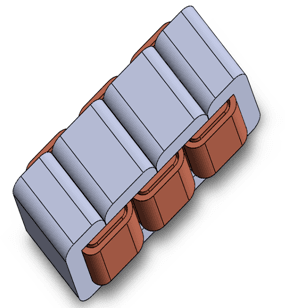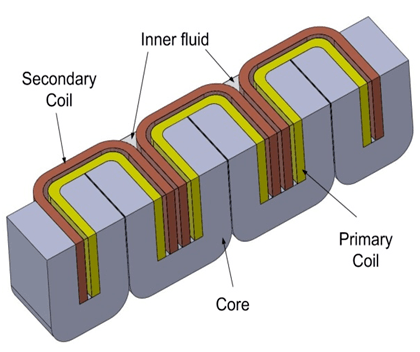Figure 3 - 3D Model of transformer

## Study

The AC Magnetic module of EMS coupled with thermal analysis is used to compute and visualize magnetic fields and thermal results. These fields are typically caused by surges in currents or voltages. This type of analysis can be linear or non-linear. It also addresses eddy currents, power losses and magnetic forces. After creating an AC Magnetic study and thermal analysis coupling in EMS, four important steps shall be followed: 1 - apply the proper material for all solid bodies, 2- apply the necessary boundary conditions, or the so called Loads/Restraints in EMS, 3 - mesh the entire model and 4- run the solver.

## Materials

In the AC Magnetic  analysis of EMS,  the whole properties of material are needed (Table 1).

 Components / Bodies Material Relative permeability Conductivity (S/m) Inner Coil / Outer coil Copper 0.99991 57e+006 Outer Air / Inner Air Air 1 0

Table1 - Table of materials

The used material in the core is a Laminated Steel (Figure 4).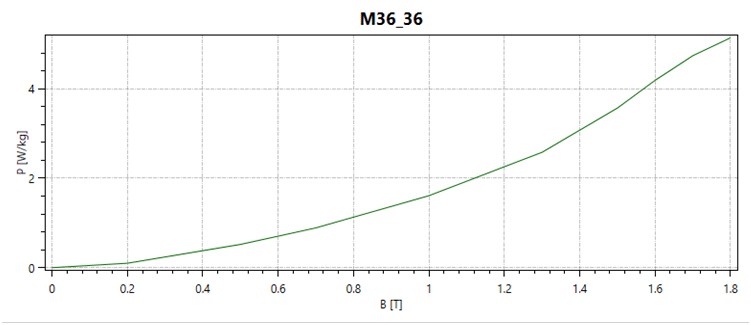Figure 4 - Core's Material properties

## Electromagnetic Input

In this study, 3 primary coils and 3 secondary coils (Table 2) are applied. The AWG is specified in EMS coil definition, it's, in this case, 38.06150 and the wire diameter of the coil is 0.10001348 mm.

 Name Number of turns Magnitude Phase Wound Coil 1 (primary) 300 1 A 0 Wound Coil 2 (primary) 300 1 A 120 deg Wound Coil 3 (primary) 300 1 A 240 deg Wound Coil 1 (secondary) 300 0 A 0 Wound Coil 2 (secondary) 300 0 A 120 deg Wound Coil 3 (secondary) 300 0 A 240 deg

Table 2 -  coils information

## Thermal Input

We need to add all air bodies as convection input

## Meshing

Meshing is a very crucial step in the design analysis. EMS estimates a global element size for the model taking into consideration its volume, surface area, and other geometric details. The size of the generated mesh (number of nodes and elements) depends on the geometry and dimensions of the model, element size, mesh tolerance, and mesh control. In the early stages of design analysis where approximate results may suffice, you can specify a larger element size for a faster solution. For a more accurate solution, a smaller element size may be required.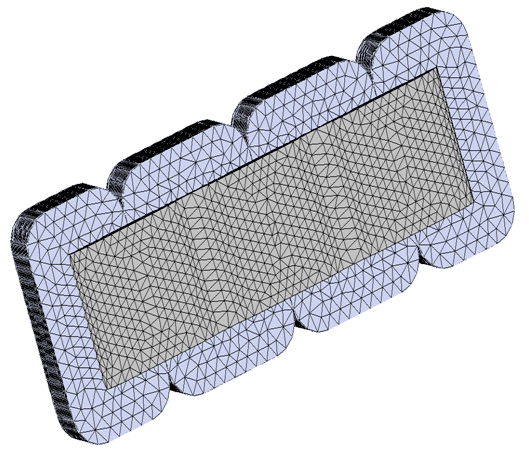Figure 5 - Meshed Model

## Results

After running the simulation of this example we can obtain many results. AC Magnetic Module coupled with thermal analysis generates the results of : Magnetic Flux Density (Figure 6,7), Magnetic Field Intensity, Applied Current Density (Figure 8),  Force density, Losses Density (Figure 9, 10) and a results table which contains the computed parameters of the model (Inductance, Current, Induced voltage, Losses etc ..) (Table 1), the electromagnetic forces . In addition to the electromagnetic results thermal results are also obtained: Temperature (Figure 11) , Temperature Gradient, Heat Flux (Figure 12).

In the results table (Table 1) we can find the induced voltage of the six coils.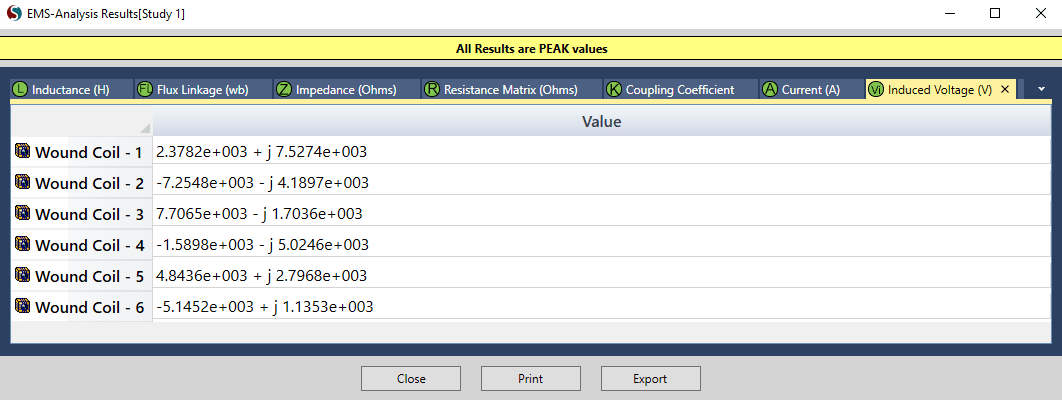Figure 6 - Results Table

EMS offers the possibility of many types of plot. Below we can observe fringe and vector plot of the magnetic flux density.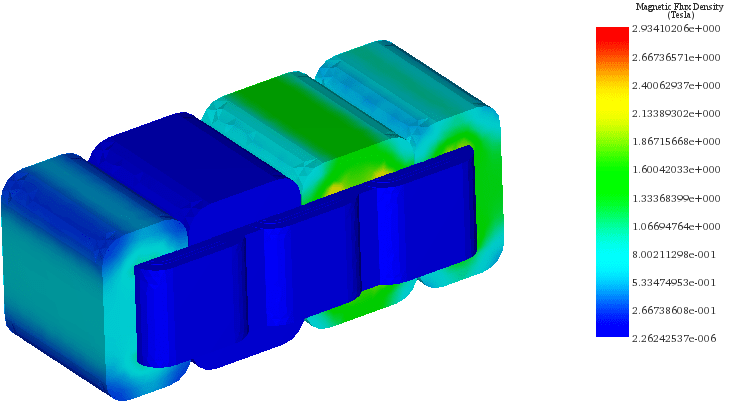Figure 7 - Magnetic Flux Density in the transformer, Phase 0 deg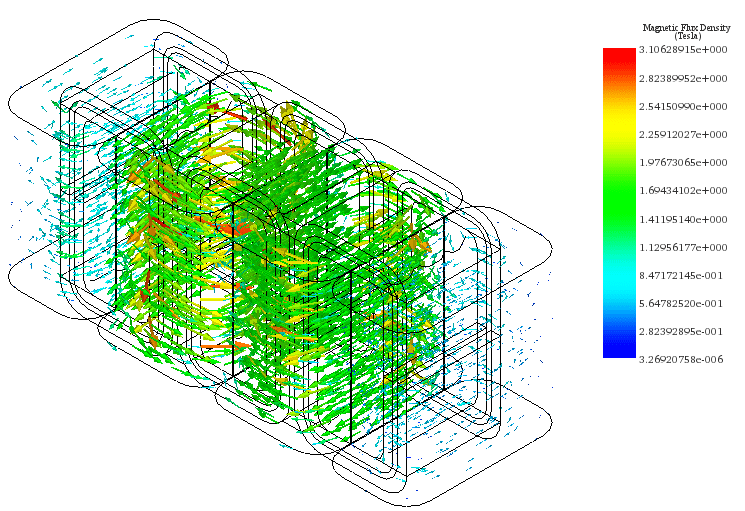Figure 8 - Magnetic Flux Density in the core of the transformer, Phase 0 deg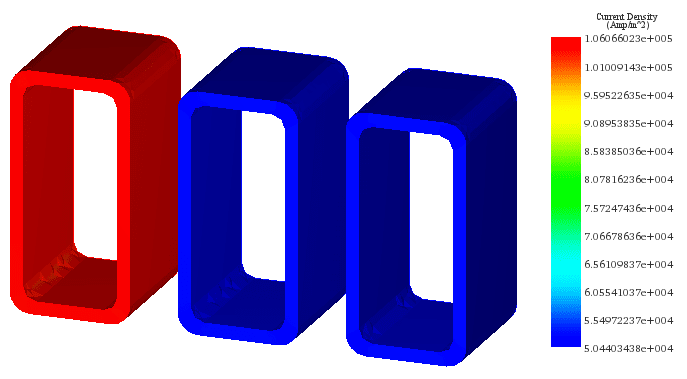Figure 9 - Applied Current Density, Phase 0 deg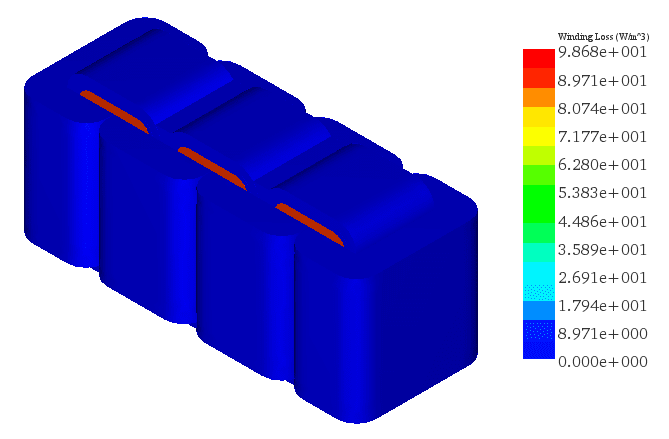Figure 10 - Winding Losses in primary coils, line plot'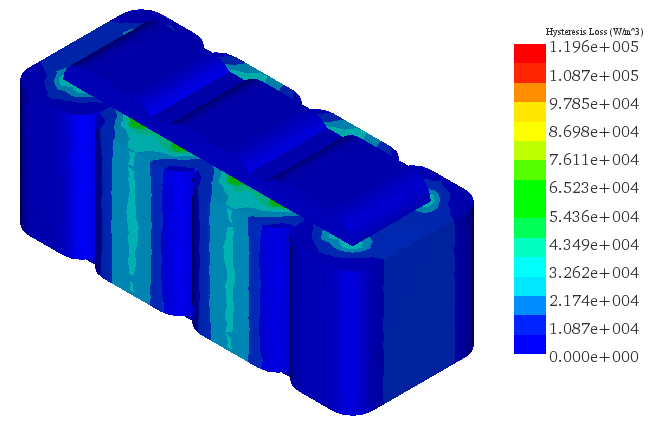Figure 11 - Hysteresis Loss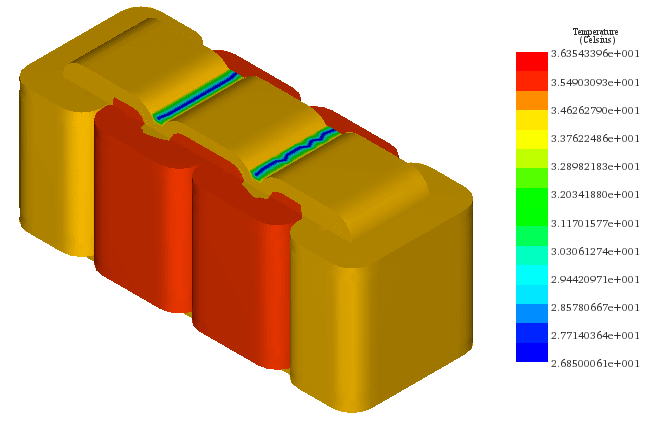Figure 12 - Temperature in the transformer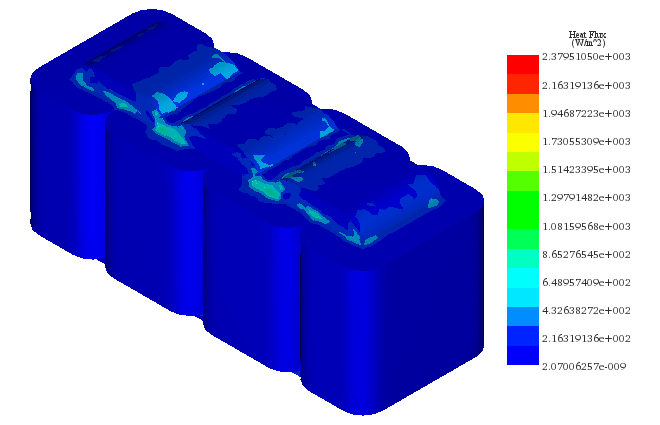Figure 13 - Heat Flux

## Conclusion

An electromagnetic analysis of the transformer model is carried out, and the results are recorded in an EMS report. The applied current density in each coil, the magnetic flux density, the magnetic field intensity, the resulting eddy current distribution, and other electromagnetic parameters are presented in the report. A steady state thermal analysis is also carried out. Using EMS, additional runs can be carried out using alternative geometric and/or material configuration to reduce the temperature rise and thus increase the transformer’s reliability and lifetime.